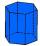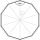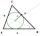# Glass

How many glass are needed to produce glass with base regular 5-gon if one base triangle in the base is 4.2 square cm and the height is 10 cm?

Result

S =  195.685 cm2

#### Solution:Leave us a comment of example and its solution (i.e. if it is still somewhat unclear...):

Showing 0 comments:Be the first to comment!#### To solve this example are needed these knowledge from mathematics:

See also our trigonometric triangle calculator.

## Next similar examples:

1. Holidays - on poolChildren's tickets to the swimming pool stands x € for an adult is € 2 more expensive. There was m children in the swimming pool and adults three times less. How many euros make treasurer for pool entry?
2. KLMN trapezoidThe KLMN trapezoid has bases KL 40cm and MN 16cm. On the KL base is point P. The segment NP divides the trapezoid into units with the same area. What is the distance of point P from point K?
3. OfficeOffice building was built in the shape of a regular hexagon inscribed in a circle with a radius of 12 m. The height of the walls is 7m. How much CZK cost plastering the walls of the building, if per 1 m square cost CZK 400?
4. ReflectorCircular reflector throws light cone with a vertex angle 49° and is on 33 m height tower. The axis of the light beam has with the axis of the tower angle 30°. What is the maximum length of the illuminated horizontal plane?
5. Regular triangular prismCalculate the surface area of body of regular triangular prism, when the length of its base edge is 6.5 cm and height 0.2 m.
6. High wallI have a wall 2m high. I need a 15 degree angle (upward) to second wall 4 meters away. How high must the second wall?
7. Isosceles right triangleContents of an isosceles right triangle is 18 dm2. Calculate the length of its base.
8. Trapezoid MO-5-Z8Trapezoid KLMN has bases 12 and 4 cm long. The area of triangle KMN is 9 cm2. What is the area of the trapezoid KLMN?
9. Regular n-gonIn a regular n-angle polygon the internal angle is 144 degrees. Find the number n indicating the number of sides of this polygon.
10. Center traverseIt is true that the middle traverse bisects the triangle?
11. TreeHow tall is the tree that observed in the visual angle of 52°? If I stand 5 m from the tree and eyes are two meters above the ground.
12. Sss triangleCalculate the area and heights in the triangle ABC by sides a = 8cm, b = 11cm, c = 12cm
13. Angles in triangleThe triangle is ratio of the angles β:γ = 6:8. Angle α is 40° greater than β. What are the size of angles of the triangle?
14. Triangle 42Triangle BCA. Angles A=119° B=(3y+14) C=4y. What is measure of triangle BCA=?
15. Inscribed circleThe circle inscribed in a triangle has a radius 3 cm. Express the area of the triangle using a, b, c.
16. Reference angleFind the reference angle of each angle:
17. Theorem proveWe want to prove the sentense: If the natural number n is divisible by six, then n is divisible by three. From what assumption we started?